## Find the midpoint of PQ with endpoints P (-4, 3) and Q (4,-1) . Then write an equation of the line that passes through the midpoint and is p

Question

Find the midpoint of PQ with endpoints P (-4, 3) and Q (4,-1) . Then write an equation of the line that passes through the midpoint and is perpendicular to PQ. This line is called the perpendicular bisector

in progress 0
6 months 2021-07-29T01:05:32+00:00 1 Answers 7 views 0

## Answers ( )

Equation of the line  that passes through the midpoint and is perpendicular to PQ is   2 x – y +1 = 0

Step-by-step explanation:

Step(i):-

Given points are P( -4 ,3 ) and Q ( 4,-1)

Mid -point of PQ

=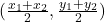=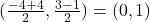Step(ii):

Slope of PQ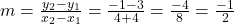The slope of the line is Perpendicular to PQ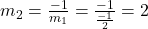Equation of the line  that passes through the midpoint and is perpendicular to PQ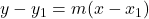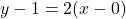2 x – y +1 = 0